﻿ Computer Simulation of the Polymerizable Oxide Melts Nanostructure Using the Descriptor-Graph Model

### Computer Simulation of the Polymerizable Oxide Melts Nanostructure Using the Descriptor-Graph Model

Voronova L.I, Grigorjeva M.A, Voronov V.I., Trunov A.S.OPEN ACCESSPEER-REVIEWED

## Computer Simulation of the Polymerizable Oxide Melts Nanostructure Using the Descriptor-Graph Model

Voronova L.I1, 2,, Grigorjeva M.A3, Voronov V.I.1, Trunov A.S.2

1National Research University Higher School of Economics, Moscow, Russia

2Russian State University for the Humanities, Moscow, Russia

3National Research Centre “Kurchatov Institute”, Russia

### Abstract

The paper describes the method for modeling of nanostructure polymerizable multicomponent oxide melts, which can be used for systems of type Me2O-SiO2 (Me = monovalent cation), with the results of a molecular dynamics simulation as input. The models of the short-range and medium-range orders taking into account dual behavior of monovalent alkali metal cations able to form stable groups with oxygen atoms are built. The melt structure is described with help of heterogeneous descriptors which are constructed using the polymer models and molecular dynamics results. The model is a heterogeneous graph which is built with gradually increasing of mapping levels (from selection heterogeneous graph vertices associated with individual particles, to forming connected components of vertices corresponding polyanionic complexes and rings in the melt. Quantitative calculations of the structure associated characteristics are carried out using the distribution function of graph vertices. We have modeled nanostructure and studied polymerization processes in the system SiO2-Na2O in the range of five compositions by the above method. In particular, we calculated the radial and angular distribution, the distribution of the coordination numbers, the bond lengths, the mole portions of different types of oxygen atoms, the complex anions in the model system taking into account sodium ions, the proportion of flat rings in polyanionic complexes, as well as the average connection factor. The obtained results give a satisfactory agreement with the characteristics in the range having experimental data. A number of results the structure modeling has a scientific novelty and practical significance.

### At a glance: Figures

123456
Prev Next

• L.I, Voronova, et al. "Computer Simulation of the Polymerizable Oxide Melts Nanostructure Using the Descriptor-Graph Model." Materials Science and Metallurgy Engineering 1.1 (2013): 1-12.
• L.I, V. , M.A, G. , V.I., V. , & A.S., T. (2013). Computer Simulation of the Polymerizable Oxide Melts Nanostructure Using the Descriptor-Graph Model. Materials Science and Metallurgy Engineering, 1(1), 1-12.
• L.I, Voronova, Grigorjeva M.A, Voronov V.I., and Trunov A.S.. "Computer Simulation of the Polymerizable Oxide Melts Nanostructure Using the Descriptor-Graph Model." Materials Science and Metallurgy Engineering 1, no. 1 (2013): 1-12.

 Import into BibTeX Import into EndNote Import into RefMan Import into RefWorks

### 1. Introduction

The oxide melts are disordered strong-interacting polymerized systems (potentials contain short-range and long-range Coulomb contributions). One of the basic problems of physical chemistry of oxide melts is the exploration of structure and physical-chemical properties correlations.

The absence of the sequential analytical theory of slag melts and difficulties of their experimental study stimulated active computer modelling of these materials. The results of computer experiments are used for design of materials with required properties.

Method of molecular dynamics (MD) has widely spread among computer methods used for study of strong-interacting systems of many particles. This method practically "of the first principles" allows obtaining various physical-chemical properties. The adequacy of modelling results is defined by accuracy of used mathematical models.

Since the success of molecular-dynamic (MD) simulation of silica carried out by Woodcock et al.  there have been many attempts at MD simulations of oxide systems in the form of crystals, glasses and melts.

A number of authors (B.J.Alder , R.L.Mozzi  A.N., R.W.Hockney , Soules T.F , Mitra S.K  and others) suggested methods of modeling systems with potentials containing short-range and long-range (Coulomb) contributions. As the Coulomb term tends to zero only at infinity and also it gives a deep minimum on the potential curve, these so-called strong-interacting systems require special accounting techniques (Ewald summation) for long-range Coulomb interaction .

In the case of silicate glasses, intensive efforts have been made during the last two decades to probe their microscopic properties by means of computer simulations The application of these methods in the high-tech computer programs ( , Belashenko D.K. [9, 10], L.I. Voronova L.I. , Hong, N.V , Mountjoy, G , Gel'chinskii, B.R , etc.) allows to study the system of 103-104 particles and to obtain the averaging structural characteristics of short-range order and some thermodynamic parameters.

A number of last papers studies containing monovalent and divalent metal cations (Na, Ca), in particular S.Ispas , Sasaki Y. , Clark T.M. , , Takada A. .

Silica and binary sodium silicate oxide glasses are of important relevance in many technology fields such as electronics, metallurgy, ceramic materials, etc. In recent decades they have attracted attention of researchers because of their practical importance.

A number of authors have successfully applied classical molecular dynamics simulations combined with first-principles calculations of NMR parameters for research local structural features, such as bond angles and bond lengths, coordination numbers (T.Charpentier , A.Pedone , F.Angeli , S.Ispas .

In the prevailing number of MD-experiments number of particles in the model cube does not exceed 1-2 thousands that gives max cube size about 2 nm, and the structural features of the study limits the short-range order.

With increasing number of particles in the model cube up to hundreds of thousands possibility appears to investigate the structural polyanionic complexes with sizes tens of nanometers.

Such MD packages as SageMD2 , HyperChem , XMD , MD-SLAG-MELT  and others allow modeling the systems of 106 particles. The most programs provide high-speed parallel computing.

Usually as a result of the MD experiment, files of coordinates and velocities several gigabytes are received and the radial distribution functions, some thermodynamic parameters (temperature, pressure, heat capacity) and diffusion coefficients are calculated based on these data using statistical methods. As a rule these characteristics are averaged over the volume and don’t give information about specific formations in the melt nanostructere.

In the oxide melts simulation, it is necessary to take into account that their features (the higher values of viscosity, the character of electricity and heat conduction, the exponential dependence of the transport coefficients on temperature) are related to the nanostructural heterogeneity and slowly developing processes that take place between the polyanionic complexes containing hundreds or thousands particles with ion-covalent bonds.

Namely the presence of large structural complexes determines the specific character of these parameters in the liquid state and their temperature dependences.

We use results of molecular-dynamic (MD) simulation with applying statistical-geometrical methods to investigate system structure with different detailing levels (short-range order, nanostructure, micro-inhomogeneity), to define features of short-range and medium-range structure, to pick out characteristics of stable clusters and regularities of their relative location.

The descriptor-graph model presented in this paper uses some basic concepts of structural organization in oxide glasses. In particular Zachariesen's “random network” model , Greaves “modified random network” model  and Esin polymer theory approaches , according to which the oxide melt is composed of different types of particles with different links. Network-forming cations form stable clusters with oxygen atoms, i.e. elementary structural groups (ESG), which are combined into polyanionic complexes of varying complexity. They can form a grid under specific conditions. Adding the modifier cations leads to the grid’s destruction, which drastically affects the physical-chemical properties of the melt.

Using the developed descriptor-graph method we find different complexity groups in the melt with various characteristics, such as a number particles of different types included in a complex, a total number of particles in a complex, sum of cations-network-formers in a complex, sum of atoms of oxygen(total, non-bridging, bridging) in a complex. Then we receive the complexes distribution functions (CDF), i.e. complex anions portions on described characteristic parameters. In addition lifetimes of different type complexes can be calculated and portions of different type oxygen atoms, portions of plain rings, system polymerization degree and constant.

### 2. Modeling Method

The descriptor-graph method developed by the authors has been realized as a module of the research-information system (RIS) with remote access «MD-SLAG-MELT» [27, 28]. The resource contains software complex combining a number of computer programs that implement the mathematical models of the structure and properties of multicomponent oxide melt [32, 33, 34]. A class of structural models is shown in Figure 1.

The base of these models is the molecular dynamic (MD) model which describes the motion of particles in the model cube. MD-results are presented in large arrays containing the coordinates of particles in three dimensions. Models of physical-chemical properties and the structure model operate on their basis, so the class of the structure’s models significantly expands the characteristics obtained in the MD- simulation of the oxide melts.

Figure 1. The classes of the models for the program complex Nano-MD-Simulation

It’s known that monovalent alkali cations (Na, K, Li) show a dual behavior and unlike others, can form stable groups with oxygen atoms. In particular, the sodium ion in the glass is in the structural groups Si-O-Na and can be connected to non-bridging oxygen.

Practically there aren’t free alkali metal oxides in alkali silicate, borate or phosphate melts, because direct thermodynamic experiments show their negligible activity in melts, and come to the conclusion that the alkali metal oxide is strongly associated with net-forming oxide .

An alkali metal atom, joining the oxygen atom belonging to the coordination polyhedra, changes its role in the structure of the melt and turns it into a non-bridging atom, giving rise to heterogeneity in the structure of the oxide systems, which are required the additional options for describing.

For these reasons the actual task is to develop a method for modeling the middle order structure of the oxide melt, taking into account its heterogeneity.

The developed method of the melt structure modeling is described in the terms of heterogeneous graph network, with an incremental increasing the mapping complexity, from the selection of diverse graph vertices, describing the investigated particles, to forming the connected components of the vertices that describe the polyanionic structure complexes in the melt, and quantify assessment of depending on the structure data.

Stages of building a class of structural models:

1. construction of the graph vertices sets model;

2. the model of the stars construction;

3. formation of connected graphs;

4. search of the plane rings;

5. the model of the structure-sensitive properties.

Investigated oxide melts are multicomponent. Therefore, to describe its heterogeneous structure, on the first stage, each particle in the model cube is associated with a descriptor d that contains a collection of heterogeneous parameters. Each parameter characterizes the structure from different points of view - in terms of the polymer model, and in terms of molecular-dynamic experiment results. Descriptor parameters d are shown below.where type is the type of the vertex, kmax - maximum allowed vertex valence, kcurr - current valence of the vertices, i is the ordinal number of vertices, r - coordination sphere radius, kf is the scales factor, s - aggregator, describing the acceptable types of adjacent vertices .

These parameters are used to transfer the notation of polymer theory to the notation of graph theory (Figure 2).

Structure-forming, modifying and homogeneous vertices which are corresponded to the certain types of atoms of the model system are determined in the graph model. Table 1 shows the values of the descriptor corresponding to the current sets. The subsets which determine the quantity of adjacent vertices, or their valence are allocated for modifying and homogeneous subsets of vertices. For example, uniform vertices can be divalent, monovalent or free, and modifying vertices can be isolated and hanging.

#### Table 1. Descriptor’s Values for Set of Vertices

Building stars for all structure-forming and modifying vertices on the second stage are based on the selected sets of vertices. Theoretically the stars determine elementary structural groups (ESG) in the melt. In the model cube for each structure-forming and modifying vertex defines the star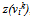, on the ends of which only homogeneous vertices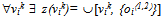can locate and the number of adjacent vertices mustn’t exceed the maximum valence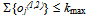and they should locate within the radius of the first coordination sphere r (Figure 3).

The obtained data can be used to calculate important characteristics of the short-range order’s structure such as coordination numbers, bond angles and radial distribution functions. These parameters are sufficiently informative and allow make comparisons with physical experiments. However, they don’t contain information about the features of the nanostructure materials, because they are averaged over the entire volume.

In the 3rd stage the average-range order’s structure is analyzed and it’s involved the processes modeling of the creation and destruction of polyanionic groups of varying complexity. The complex in the graph model is described by the connected graph, which consists of modifying the structure-forming vertices stars. Two stars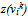and z(vjk) are connected in coincidence homogeneous bivalent vertices om2 = on2, where om2, on2z(vjk). The complex can be uniform and non-uniform, and is described by the adjacency list of connected graph verticeswhereFurther modeling associated with the research of the structural features of complexes is complicated by the presence of a large number of connected elements, which are explained by the presence of large polyanions in the melt. To reduce the number of investigated elements in the graph model the concept of a "second level" connected graph is introduced. Its vertices are the stars. This can significantly reduce the dimensionality of the complexes, remains its structure.

The homogeneous complex (Figure 4) is a compound of stars of vertices belonging to the same structure-forming set. The heterogeneous complex (Figure 5) is a compound of stars of vertices belonging to different sets of structure-forming.

G( [Ring(v1k), z(v2k),…, Ring(vNk)]), where ki kj, ri rj and ∀Ring, Ring(vjk) vm2 Ring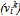= vn2 Ring(vjk)

Polymer theory postulates that the gaps of polyanionic grid occur in the structure of oxide melts under the specific conditions. The developed graph model, on the its 4th stage allows to search the net gaps on the "second level" graphs by the presence of simple chains (Figure 6), which represent the minimal sequence of distinct connected vertices, the initial and final elements are the same.

The classes of multilevel graph models, described above, allow get the detailed evaluation of the nanostructure numerical characteristics. The structure-sensitive properties model is built on the data and it connects physical and chemical properties with the structural features of graph models .

The model of structure-sensitive properties gives 2 blocks of parameters: the distribution function of graph vertices and scalar structural characteristics.

To analyze the complexes distribution a new descriptor dG, applied to a set of vertices, is defined. It is dG = [Type, N, Nvk, No, No2, No1, NRing, τ ], where Type is the type of a connected graph, N – the total number of vertices, Nvk the number of the “second level” vertices, No – the number of homogeneous nodes, No2 the number of homogeneous divalent vertices, No1 – the number of homogeneous suspension tops, NRing the number of the "second level" vertices belonging to the simple rings, τ is the lifetime of a connected graph. The distribution function is constructed for each descriptor’s item. These functions allow us to estimate the dynamics of the complex process and to investigate the behavior of different complexity atom groups in the melt.

Distribution function of the connected graphs vertices on base of dG elements

We can write formula for the complex vertices distribution: f(dG) = ∑ τi / n, where f(dG) is the portion of complexes with the same numerical value of dG element, n the number of configurations, τi the lifetime of i-complex, and the sum is taken up to the number of complexes with the same numerical value of the descriptor.

Distribution function of the complexes lifetime which depend on the dG element’s value can be represented as τ̅(dG) = ∑ τi / i, where τ̅(dG) is the average complex lifetime, τi the lifetime i of the complex with the same characteristic parameter; the sum is taken over all complexes with the same characteristic parameter.

Scalar structural characteristics

In addition to the distribution functions, the model of structure-sensitive properties allows to get a number of thermodynamic parameters that are widely used in practice. These characteristics are used to describe the polymerization processes in the melts, which are listed below.

1. The portions of homogeneous vertices in a model system:, where n is the total number of modeling configurations and the sum is taken over all configurations. In the polymer theory notation the portions of the vertices are thomogeneous portions of oxygen ions of different types: bridging, non-bridging and free.

2. The portions of the planar rings in the complex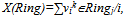where the sum is taken over all complexes. The portion of planar rings characterizes the gaps in the network structure of the oxide melt.

3. In the scientific literature, the glass structure is characterized by the average factor of the connection structural framework Y, which is the average number of bridging oxygen ions in the polyhedron formed by network-forming ion with oxygen. Y=(∑Iiki - ∑Ij)/∑Ii where ∑Ii is the sum of atoms with more than one number of connections, ∑Ij is the sum of atoms of links equaling 1 (alkali metals and halogens); ki means valence or coordination number. When displaying the polymer model to graph-theoretic one, average connection factor Y can be expressed through the ratio of the doubled quantity of homogeneous bivalent tops in modeling system to the quantity of stars of the "second level", or a certain type of structure tops.These formulas can be used to enhance the characteristics of polymerizing oxide systems.

4. The degree of polymerization can be expressed as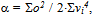where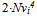is the number of structure-forming vertices.

5. The polymerization constant can be expressed by the relation, whereis the proportion of different types of homogeneous vertices.

6. The activity of the network-forming and modifying oxide in the system is expressed through the polymerization constant and the proportion of the structure and homogeneous isolated vertices: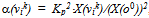, and for modifying oxide it’s expressed through the portion of homogeneous isolated vertexes α(mik) = Xo0.

### 3. Computational Procedure

We have applied worded approach to binary system SiO2 Na2O, which is widely used in the metallurgical and glass industry and represents important simplified model system for assessing new approaches for structural investigations. For the system modeling it was used the RIS [27, 28].

The system was simulated in the range of six compositions: X(SiO2) =0,4; 0,43; 0,5; 0,63; 0,74; 0,8, where X is the mole portion of the component in brackets.

Table 2 shows the input parameters of the MD simulation: they are melting temperature (Tmelt), modeling temperature (Tmod), density of the system (ρ), molar volume (Vμ).

The model compositions density of SiO2 Na2O system was calculated by the molar volumes of solid phases with an increase of 10%.

Tmod of the compositions were selected in excess of 30 to 50 degrees above the experimental Tmelt of the composition according to the state diagram of the system . We have assumed that selected composition’s set would provide full information to smooth areas as well as to singularity of the phase diagram.

Parameterization of the potential functions for the interparticle interaction in the MDsimulation was carried out with the ion-covalent model (ICM) applied for systems, containing stable long-living clusters with a high share of covalent connections. The pair spherical-symmetrical long-range Coulomb interaction, the two- and three-particle covalent interactions are taken into account in ICM.

The particles of modeling system – cations network-formers(CNF), cations-modifiers (CM) and anions of oxygen(O) have the following attributes in ICM: mi -mass, qi–effective charge, σi – effective radius, ri - radius-vector, vi - velocity; besides there are sets: d0 – CNF-O bond length, θ0- equilibrium O-CNF-O bond angle and force constants: αkit – two-particle covalent interaction, βkit – three-particle covalent interaction.

The potential form for every particle is defined by particle’s attributes and its membership in an elementary structural group (ESG), so called “the belonging condition”. Since any particle has a charge as an attribute, the Coulomb interaction is described by a pair ionic sphere-symmetric potential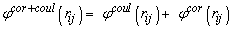(1)

that has terms in the Pauling's form,

φcor+coul(rij)=qiqje2/4πεε0rij2[1+sign(qiqj)/(n+1)*((σij)/rij)n],

where rij is a particle distance, e is the electron charge, n is repulsive curve steepness parameter.

The belonging condition: In case the particle belongs to a "regular" ESG which contains oxygen atoms in number corresponding to atom network-former valence, potential (1) is supplemented by two- and three-partial covalent contributions:

two-particle potential:(2)

three-partial potential: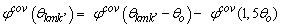(3)

where m is the index of silicon atom in centre of ESG; k and k' are indexes of oxygen atoms in ESG; θkmk is O-CNF-O equilibrium angle; 1.5d0, 1.5θ0 are maximum covalent two- and three-particle forces action radius and angle respectively.

The introduction the last terms in the Equations (2) and (3) ensures the continuity of the potential functions when adding the covalent interactions.

Covalent contributions are described in the Keating's approximation :(4)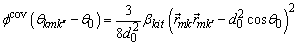Potential energy φm of covalent interaction of belonging to m-elementary complex particles: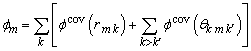Total potential energy of the simulated system, considering the covalent interactions inside an elementary complex: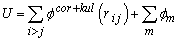Table 3 shows the parameters of the potential functions for the main types of interactions in the system. The parameters were determined using the semiempirical quantum-chemical method MNDO, based on the electronic structure computation in our earlier papers [37, 38].

#### Table 3. Parameters of Potential Functions for MD Simulation SiO2-Na2O System

Molecular dynamics simulations are carried out on the models consisting of 10000 particles approximately and vary slightly depending on the composition.The simulation has been performed in a cubic cell where the periodic boundary conditions were applied. Particles insert in a cubic box according to the glass composition and the experimental melt density, with parameters given in Table 2. The initial configuration is generated by placing all particles like the crystal lattice of SiO2 and then some atoms have been replaced by sodium atoms randomly and the number of oxygen atoms was corrected. The Table 4 contains data about the number of atoms and molecules each type, depending on the composition

#### Table 4. Number of particles of each type in the model systems

The systems were initially equilibrated at 6000K for 10 ps in the NVT ensemble to ensure suitable mixing of the melt and then cooled down to Tmod, through 50 temperature points, when the velocity of particles was scaled by (6000- Tmod)/50 and during 0,5ps steps the system was stabilized on each temperature point.

When Tmod is achieved the system enters the thermo-stabilization phase - the program tests fluctuations of temperature on 0,15ps. If the fluctuations have exceeded 23К, the system is stabilized the next time period. If the average fluctuation does not exceed 23К, the system goes into a thermodynamic equilibrium phase, which typically lasts 10 ps. The thermodynamic equilibrium phase contains twenty macro-steps, each lasting 0,5 ps (1000 micro-steps), that allows to realize statistical processing of the modeling data.

The equation of motion were integrated using the Beeman algorithm with a time step 0.5 fs.

### 4. Results and Discussion

As a result of series of computer experiments the structural, thermodynamic and transport properties were obtained. These properties were entered into a database on the application server http://nano-md-simulation.com . Additionally, the binary files containing the coordinates and velocity for each time step were formed. These files allow the parallel processing Big data using statistical and geometric methods.

The descriptor-graph method of the structure modeling uses values shown below for the heterogeneous vertices in the graph network:

1) for the cations of silicon (Si)

Type=S, kmax=4, r=1.6, kf=1.45, s=[O,{[Type=M, kmax=1]}];

2) for the cations of sodium (Na)

Type = M, kmax=1, r=2.4, kf=1.45, s=[O,S];

3) for the cations of oxygen (O)

Type = O, kmax=2, s=[S,M].

After MD-simulation partial radial distribution functions gαβ(r) were obtained for all particles types depending on the composition. gSi-O(r) for all compositions have a high and well-resolved first peaks and clearly defined second ones; gNa-O(r) and gO-O(r) have lower and broader first peaks and "smeared" second ones. The character of the RDF has not practically changing depending on the composition.

Characteristics of a short-range order structure were calculated, in particular the coordination numbers (CN) (Figure 7), bond lengths(L) (Figure 8) and valence angles distributions (Figure 9).

The average distance for the Si-O is 0.163 nm (which is close to the values of the average bond length for the pure oxides [31, 36]), for Na-O value lays in the range of 0,236-0,248nm, that correlates with the published data [15, 35, 23]. Medium distances for the other types of particles are practically independent of the composition also, and they vary by 2-3%. The exception is the average distance of Na-Na, which varies up to 12% (2,64-2,98A), due to the high mobility of sodium atoms, as well as their ability to join to the silicon-oxygen complexes.

The coordination number of Si-O throughout range of compositions remains constant and is equal to four that speaks about the presence of tetrahedral complexes even at low mole fraction of SiO2.

The coordination number of Si-Si with increasing mole fraction of SiO2 from 0.4 to 0.8 gradually increases from 2.3 to 8.26. In pure SiO2 the value is 10. Since this is not the first coordination sphere, the increase in the CNSi-Si says about the growing regulation of the complexes in the melt and grid forming. Larger values CN for Si-Na and Na-Na are associated with a lack an explicit first peak on the RDF these types of atoms. It’s indirect confirmation of permanent migration of Na atoms in the melt. Lowering the values these CN at high mole fraction of silicon oxide is associated with total amount of Na atoms decrease in the model cube.

More information about the particle distribution in the complexes and the number of different types of complexes in the melt can be obtained using descriptor- graph model and the results will be shown below.

Along with the RDF the angular distribution functions (ADF) of all types of particles were calculated. Figure 9 shows the ADF for the valence angles and angles between bonds. ADF have a similar kind regardless of the composition. The observed increase in amount of both the valence angles (O-Si-O), and angles between bonds (Si-O-Si) with increasing mole fraction of SiO2 explained by an increase of the silicon atoms number in the melt (Table 4).

The average angles were calculated based on the ADF. Their values are correlated with the suitable coordination numbers. The constancy of the coordination number of Si–O leads to the constancy of the mean O–Si–O angle both for the acid compounds with a large amount of SiO2, and for the base compounds with a few network-forming atoms.

The average value of the valence angle 109o corresponds to the sp3-hybridization and is independent of whether there is a melt-dimensional network or a set of individual elementary tetrahedra SiO4-.

Angles of Si–O–Si, i.e. the angles between adjacent structural groups are in the range of 120 to 180° The average value of the Si–O–Si angle is approximately 144°. This value with high content of SiO2 is correlated with the experimental data [35, 36].

The above structural the short-range order parameters are sufficiently informative and allow making a comparison with the natural experiment. However, they don’t provide information about the features of the nanostructure, as they are averaged over the entire volume. They say nothing about the medium- and long-range structure, which is the determining in shaping the physical-chemical properties of slag melts and provide no information about the polymerization/depolymerization processes occurring in the melt at the change its composition (e.g. increasing the molar fraction of the oxide modifier).

Below we investigate some aspects of the melt polymerization system SiO2-Na2O that are not usually extract from the dynamics trajectories using the descriptor-graph method.

More detailed information on the nanostructure of the melts was obtained from the complex anions distribution functions in a number of characteristic parameters described in Section 2 “Modeling method”.

The distribution functions of complex anions on various values descriptor’s element dG were obtained for all simulated compositions.

To compare the results the structural modeling was carried out with (Figure 10) and without (Figure 11) allowances connection of sodium atoms to non-bridging oxygen atoms in polyanionic complexes. These figures show the distribution histograms of the complex anions by descriptor’s element dG = Type, as the most indicative characteristic. On the horizontal axis there is the type of the complex, and on the vertical axis - the proportion of complexes of this type in the model cube.

The analysis has shown that adding of a large number of sodium ions (X (Na2O) > 0,5) leads to a constant renewal of polyanionic complexes because of the sodium ions migration. Moreover, the sodium atoms connect to the non-bridging oxygen atoms in complexes of small size (NSi = 1-50). Sodium atoms also join free oxygen atoms, reducing its number in the model cube to almost zero.

The structure of small polyanionic complexes with associated sodium atoms is more stable on the number of network-forming atoms than the structure of complexes without sodium atoms. However, it’s typical for the systems with a large amount of sodium.

The sodium atoms, joining non-bridging oxygen atoms in polyanionic complexes, and connecting with the free oxygen atoms, reduces the proportion of non-bridging and free oxygen atoms in the model cube as shown in the graphs in Figure 12 (a, b), which provides the distribution of free, non-bridging and bridging oxygen, depending on compound. Free oxygen concentration is reduced to zero at X(SiO2) = 0,7. Amount of the non-bridging oxygen is characterized by the convex curve, the maximum of which corresponds to the composition X(SiO2) = 0,43.

The polymerization process is observed when increasing the molar portion of silica. Elementary structural complexes SiO4 are grouped in polyanions of the increasing complexity. In the thermodynamic equilibrium phase for each temperature point there is a process of oxygen atoms sharing, destruction and restoration of polyanions. Oxygen atoms and small structure groups with 1-2 silicon atoms are constantly joining and leaving large complexes, due to the migration of sodium atoms. The process of large silicon-oxygen groups formation leads to a continuous network formation.

Low-dimensional silicon complexes which contain 1-4 network-forming atoms are dominated in the composition range X(SiO2) = 0.40.5 (Figure 13a). However, there are large polyanions with a maximum lifetime even in this area (Table 5). Although their relative portion is small, they combine from one to three—quarters of atoms in the system. The lifetime of the Si1-4 groups is also long, although there are some groups, living only a few configurations. We suppose that they are the debris of the large complexes, to which they are connecting in the end.

#### Table 5. Complexes lifetime inSiO2-Na2O system

In the compounds with X(SiO2) = 0.4, 0.43, 0.5 the portion of orthogroups SiO4 is from 33% to 50% of the total number of complexes. It indicates to the presence of orthosilicates with various modifications in the system. The compositions with X(SiO2) = 0.4, 0.43 contain Si2O7 diortogroups, which is about 22% of the total number of complexes. This indicates to the presence of pirosilicates in the system. Moreover, the composition X(SiO2) = 0.5 contains polyanions Si3 (rings) (11%) and Si4-9 (22%).

Starting with X(SiO2) = 0.63 small groups containing more than 2 silicon atoms disappear, leaving only orthogroups and a large polyanion. Therefore, starting with this composition, the system is almost completely polysssmerized and represents a three-dimensional network (Figure 13b).

Dependence on the polymerization degree and the polymerization constants of the system’s composition is shown in Figure 14 and Figure 15.

We can see that the polymerization constant decreases with the increasing amount of SiO2. When oxide is polymerized, there’s practically no ion migration inside the grid, and the number of free oxygen decreases from compound to compound.

There is an interesting feature in the investigating system - the gap in the dimension of radicals. There are complexes containing from 1 to 8 silicon atoms and complexes containing from 74 silicon atoms and above for different compounds. There are no polyanions which contain from 9 to 73 silicon atoms in the system.

Figure 16 shows the distribution of planar rings portions in the complexes. The maximum portion of rings was observed in X(SiO2) = 0.5. It indicates that large polyanionic complexes contain more than 65% of the anions in ring compounds. In the other compounds the average number of planar rings is about 30%. Analysis showed that in a large complexes (NSi> 50), planar rings may be composed of a different number of tetrahedral groups, but mostly out of 3, 4 and 6 tetrahedra.

The results of calculation of the average connection factor (Table 6) have shown that the values for the silicate glasses, as a rule, are in the range of 2 to 4. Therefore a continuous network of bridge bonds with the formation at Y = 4 is three-dimensional wireframe, with Y = 3 - dimensional layered and Y = 2 one-dimensional chain structure is formed in the glasses.

#### Table 6. Average Connection Factor Y

In the ideal case, when Y < 2, the structure won’t be a continuous frame, and will consist of chains of limited length and isolated structural fragments. Under such conditions (Y < 2) glass forming is impossible. But in the case of a mixed structure forming, consisting of the fragments with Y = 2, Y = 1, Y = 0, i.e. of infinite chains, two-term chains and isolated fragments with average values of Y, close to 2, it is quite possible to get glass, that is confirmed by the investigation.

The results obtained in modeling structures coincide with the experimental data and show that adding the sodium oxide to the quartz glass looses the glass structure due to the rupture of the Si–O bonds formed by the bridging oxygen atoms, which are connected through the neighboring coordination of tetrahedrons. During this process non-bridging oxygen atoms are formed. With the introduction of up to 33% Na2O two-dimensional structures are formed, which contain chemically bound volumetric tetrahedrons in their planes. The layers are connected by a weaker ionic interaction. Thus, sodium oxide reduces the degree of the quartz glass connectivity.

### Conclusion

The Descriptor-Graph method developed by the authors has been implemented as a module for the research-information system with the remote access «MD_SLAG_MELT». There were used expediting algorithms for this method that allowed to process large volumes of the molecular dynamic simulations results.

The melt structure is described with help of heterogeneous descriptors which are constructed using the polymer models and molecular dynamics results. The nanostructure model (medium-range order) takes into account dual behavior of monovalent alkali metal cations able to form stable groups with oxygen atoms.

To test the method we have modeled the structure of sodium silicate binary system in the range of six compounds. In particular, we calculated the radial and angular distribution, the distribution of the coordination numbers, the bond lengths, the mole portions of different types of oxygen atoms, the complex anions distributions lifetimes ones in the model system taking into account sodium ions, the proportion of flat rings in polyanionic complexes, as well as the average connection factor.

The obtained results give a satisfactory agreement with the characteristics in the range having experimental data. A number of results the structure modeling has a scientific novelty.

### References

  L.V.Woodcoock, C.A.Angell, P.Cheeseman “Molecular dynamics studies of the vitreous state: simple ionic system and silica” J.Chem.Phys., 65(4): 1565-1577, 1976.In article CrossRef  Alder B. J., Wainwright T. E. “Studies in Molecular Dynamics. I. General Method”, J.Chem. Phys., 31(2): 459-466, 1959.In article CrossRef  R.L.Mozzi, B.E.Warren “Structure of vitreous silica”. Journal of Applied Crystallography, 2 (pt 4): 164-172, 1969.In article CrossRef  R.W.Hockney, J.W.Eastwood “Computer simulation using particles”, McGraw-Hill Inc, p.540, 1981.In article  Soules T.F., Arun K.Varshneya “Molecular Dynamic Calculations of A Sodium Borosilicate Glass Structure”. J.Amer.Ceram.Soc., 64(3): 145-150, 1981.In article CrossRef  Mitra S.K. “Molecular dynamics simulation of silicon dioxide glass”, Phyl.Mag., B, 45(5): 529-548, 1982.In article  Anastasiou N., Fincham D. “Program for dynamics simulations of liquid and solid. II. MDIONS: Rigid ions using the Evald sum”. Comput.Phys.Commun., 25: 159-176, 1982.In article CrossRef  Zhang, L., Sun, S., Jahanshahi, S. “Molecular dynamics simulations of silicate slags and slag-solid interfaces”, Journal of Non-Crystalline Solids, 282 (1): 24-29, 2001.In article CrossRef  Belashchenko, D.K., Ostrovskii, O.I. “Computer simulation of small noncrystalline silica clusters”, Inorganic Materials, 38(9): 917-921, 2002.In article CrossRef  Belashchenko D.K., Ostrovskii O.I. “Computer simulation of the structure of liquid alkaline-earth metal chlorides based on diffraction data”, Russian Journal of Physical Chemistry. 77(12): 1972-1983, 2003.In article  Voronova L.I., Glubokij V.I., Voronov V.I., Grokhovetskij R.V. “Calculation of self-consistent set of potential parameters for the binary oxide melts MNDO-MD simulation”. Melts, 2(2): 66-74, 1999.In article  Hong, N.V., Huy, N.V., Hung, P.K. “The structure and dynamic in network forming liquids: Molecular dynamic simulation”, International Journal of Computational Materials Science and Surface Engineering, 5 (1): 55-67, 2012.In article CrossRef  Mountjoy, G., Al-Hasni, B.M., Storey, C. “Structural organization in oxide glasses from molecular dynamics modeling”, Journal of Non-Crystalline Solids, 357 (14): 2522-2529, 2011.In article CrossRef  Gel'chinskii, B.R., Belashchenko, D.K., Dul'dina, E.V., Lozovskii, E.P. “Computer Model for a Multicomponent Slag-Forming Mixture Melt: Relation between Its Atomic Structure and Physicochemical Properties”, Russian Metallurgy (Metally), (2):148-152, 2011.In article CrossRef  Ispas, S., Benoit, M., Jund, P., Jullien, R. “Structural and electronic properties of the sodium tetrasilicate glass Na2Si4O9 from classical and ab initio molecular dynamics simulations”, Physical Review B - Condensed Matter and Materials Physics, 64 (21): 2142061-2142069, 2001.In article CrossRef  Sasaki, Y., Urata, H., Ishii, K. “Structural Analysis of Molten Na2O-NaF-SiO2 System by Raman Spectroscopy and Molecular Dynamics Simulation”. ISIJ International, 43(12): 1897-1903, 2003.In article CrossRef  Clark, T.M., Grandinetti, P.J., Florian, P., Stebbins, J.F. “Correlated structural distributions in silica glass”, Physical Review B - Condensed Matter and Materials Physics, 70 (6): 064202-1-064202-8, 2004.In article CrossRef  Asada, T., Yamada, Y., Ito, K. “The estimation of structural properties for molten CaO-CaF2-SiO2 system by molecular dynamics simulations” . ISIJ, l48 (1): 120-122, 2008.In article  Takada A. “Molecular dynamics simulation of deformation in SiO2 and Na2O-SiO2 glasses”. Journal of the Ceramic Society of Japan, 116 (1356): 880-884, 2008.In article CrossRef  T.Charpentier, S.Ispas, M.Profeta, F.Mauri, C.J. Pickard “First-Principles Calculation of 17O, 29Si, and 23Na NMR Spectra of Sodium Silicate Crystals and Glasses”. J. Phys. Chem. B, 108(13): 4147-4161, 2004.In article CrossRef  A.Pedone, T.Charpentier, M.C.Menziani “Multinuclear NMR of CaSiO3 glass: simulation from first-principles”. Phys. Chem. Chem. Phys., 12: 6054-6066, 2010.In article CrossRef PubMed  F.Angeli, O.Villain, S.Schuller, S.Ispas, T.Charpentier “Insight into sodium silicate glass structural organization by multinuclear NMR combined with first-principles calculations”. Geochimica et Cosmochimica Acta, 75: 2453-2469, 2001.In article CrossRef  S.Ispas, T.Charpentier, F.Mauri, D.R. Neuville “Structural properties of lithium and sodium tetrasilicate glasses: Molecular dynamics simulations versus NMR experimental and first-principles data”. Solid State Sciences, 12: 183-192, 2010.In article CrossRef  SAGEMD2 http://www.sagemd.com/htmls/aboutsagemd. htmIn article  HyperChem.: http://www.hyper.comIn article  XMD (Molecular Dynamics for Metals and Ceramics).http://xmd.sourceforge.netIn article  Voronova L.I, Voronov V.I. “The Research-Information System "MD-SLAG-MELT". Certificate of state registration of computer programs № 2012615018 from 05.06.2012.In article  Program complex Nano-MD-Simulation.http://nano-md-simulation.com.In article  W.H. Zachariesen, J. Am. Ceram. Soc. 54 (1932) 3841.In article CrossRef  G.N. Greaves, J. Non-Cryst. Solids 71 (1985) 203.In article CrossRef  O.A. Esin “Polymer model of molten salts”. Journal of Physical Chemistry, 50(7): 1885-1836, 1976.In article  Voronova L.I., Grigorieva M.A. “Information model of physical-chemical melt properties developing for the research complex MD_SLAGMELT”, Interbranch information service, 2: 30-36, 2011.In article  Voronova L.I., Grigorieva M.A., Voronov V.I. “Nanostruсture computer modeling methods development for multicomponent slag melts”, Fundamental researches, 8(3): 617-622, 2011.In article  Voronova L.I, Grigorieva M.A, Voronov V.I, Trunov A.S “Program complex «MD-SLAG-MELT» for simulation of nanostructures and properties of multicomponent melts”. Melts, 2: 1-16, 2013.In article  Schultz, MM, O. Mazurin “Modern views on the structure of glasses and their properties”. Nauka, Leningrad, 1998.In article  The slag’s atlas. Reference book. Translated from the German. Edited Kulikov I.S. Moscow, Metallurgy, 208 p., 1985.In article  L.A.Trofimova, L.I.Voronova “The computer simulation of the interaction Me-O (Me=Si, B, Al) in the ionic-covalent model”. Proceedings of the Chelyabinsk Scientific Center, 2(36): 76-81, 2007.In article  L.A.Trofimova, L.I.Voronova "Design and construction of the potential curves of Si-O0 and Si-O- with the effect modifier in the system Si-O-Na". Proceedings of the Chelyabinsk Scientific Center, 2(36): 82-86, 2007.In article  Mitra S.K. “Molecular dynamics simulation of silicon dioxide glass”. Phyl.Mag. B, 45(5): 529-548, 1982.In article CrossRef  Gaskell P.H., Tarrant I.D: “Refinement of random network model for vitreous silicon dioxide”. Phil.Mag. 42(2): 256-286, 1980.In article  http://jmol.sourceforge.net.In article